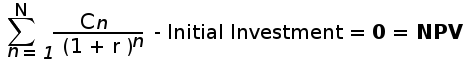# Formula for IRR calculation

IRR is the capital budgeting tool used to evaluate a desirability of a project. The IRR stands for internal rate of return for a project or investment. It's value is usually calculated in percentage. It is computed in term of NPV (Net present value). So, stating in term of NPV, IRR is the discouted rate that makes the value of NPV as 0 ( NPV = 0 ). So, the formula for IRR is same as the formula for NPV. The formula can be written as below :Where in the above formula :

N = total number of periods

n = positive integer

C = cash flow (negative or positive value)

r = internal rate of return ( or discounted rate)

NPV = net present value

Evaluating the above formula, the formula for n cash-flows can be written in simple terms as:

0 = C1/(1+r) + C2/(1+r)2 + C3/(1+r)3+ ....+ Cn/(1+r)n - initial investment

## IRR calculation with formula manually

By obtaining the IRR of several projects, we can determine which project is more likely to provide a better return. A higher IRR means that we have to discount our future cash flow by a greater interest rate, so that they equal our investment. As a result, projects that yield a higher IRR are considered to be more attractive compared to their lower yielding counterparts.

IRR can be easily calculated using Excel software and financial calculators but when you are calculating that manually then it's little tricky. We need to go through trail and error process utilizing the above formula.

As an example, lets say your are planning on investing \$10000 in a project that is expected to produce cash flow \$3500, \$4000 and \$5000 for three consecutive years. Let's calculate the IRR for this investment.

After putting value in above formula, here's how the IRR equation looks:

0 = 3500/(1+r) + 4000/(1+r)2 + 5000/(1+r)3 - 10000

We can obtain the IRR only with trail and error method. Obtaining the exact IRR value is a daunting task. So, our goal should be that what value or r (discounted rate) can be given into above equation that makes it equal (or atleast close) to 0 (NPV). Let's take the r as 0.10

0 = 3500/(1+0.10) + 4000/(1+0.10)2 + 5000/(1+0.10)3 - 10000

Now, the equation is much easier to solve. Let's simply this further.

0 = (3500/1.1 + 4000/1.21 + 5000/1.33)- 10000

In order to get NPV = 0, the value of (3500/1.1 + 4000/1.21 + 5000/1.33) must be equal to 10000 (initial investment)

0 = (3181.81 + 3305.78 + 3759.39)- 10000

0 = (10196.98)- 10000

As you can see in the above example, the equation is not equal to zero

Again using the trail-error method, we will repeat the process with value or r as 0.11

0 = 3500/(1+0.11) + 4000/(1+0.11)2 + 5000/(1+0.11)3 - 10000

Now, the equation is much easier to solve. Let's simply this further.

0 = (3500/1.11 + 4000/1.23 + 5000/1.37)- 10000

In order to get NPV = 0, the value of (3500/1.11 + 4000/1.23 + 5000/1.37) must be equal to 10000 (initial investment)

0 = (3153.15 + 3252.03 + 3649.63)- 10000

0 = (10054.82)- 10000

So at r = 0.11 the value of equation approaching near zero.

So, we can say the IRR of the above investment is 0.11x100 = 11 percent (approx.)

This is how you can apply the formula for calculating the IRR value manually.

Check: IRR Calculator Online